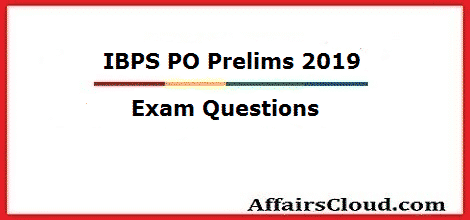#Questions asked in IBPS PO Prelims Exam 2019 – October 12

Questions asked in today’s IBPS PO Prelims Exam 2019 are listed below. If you have any more questions from the exam, then kindly share it in the comment section.#### Questions asked in IBPS PO Prelims Exam 2019 – October 12

Quant:

Approximation:

1. [(1/7.01) + (1/13.99)+(1/27.99)]× 100.04 = ?
2. 111.01-211.09+(12.01)2=?-(4.09)2
3. 3.07×14.96+(15.02)2-(11.03)2=?
4.  (14.03)+(28.02)2÷6.98+(3.96)2 = ?

Simplification:

1. 12, 129, 233, 324, ?, 467
2.  8, ?, 4, 6, 12, 30
3. 100, 97, 92, 83, 68, ?
4. 1, 2, 5, 16, 65, ?
5. 0.75, ?, 3, 6, 12, 24
6.  8, 737, 252, 568, ?, 477

1.  x2-13x=30=0; y2-3y+2=0
2. 4x2+5x+1=0; 2y2+5y+3=0
3. (x-8)2=0; y2-18y+81=0
4. x2+58=202; y2-169=0
5. x2+10x+21=0; y2+17y+72=0

**Questions are collected purely based on the feedback from aspirants. If you find any error then kindly report it.

Click Here To View IBPS PO Prelims 2019 Exam Review –  October 12

Click Here To View IBPS PO Prelims 2019 Exam Review –  October 19